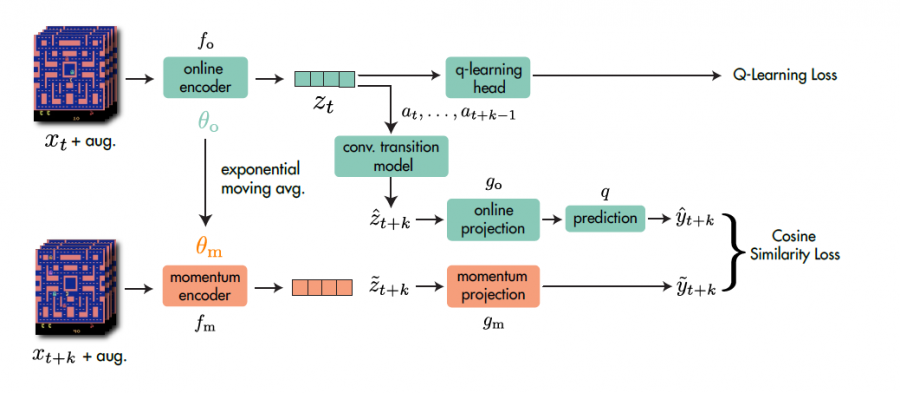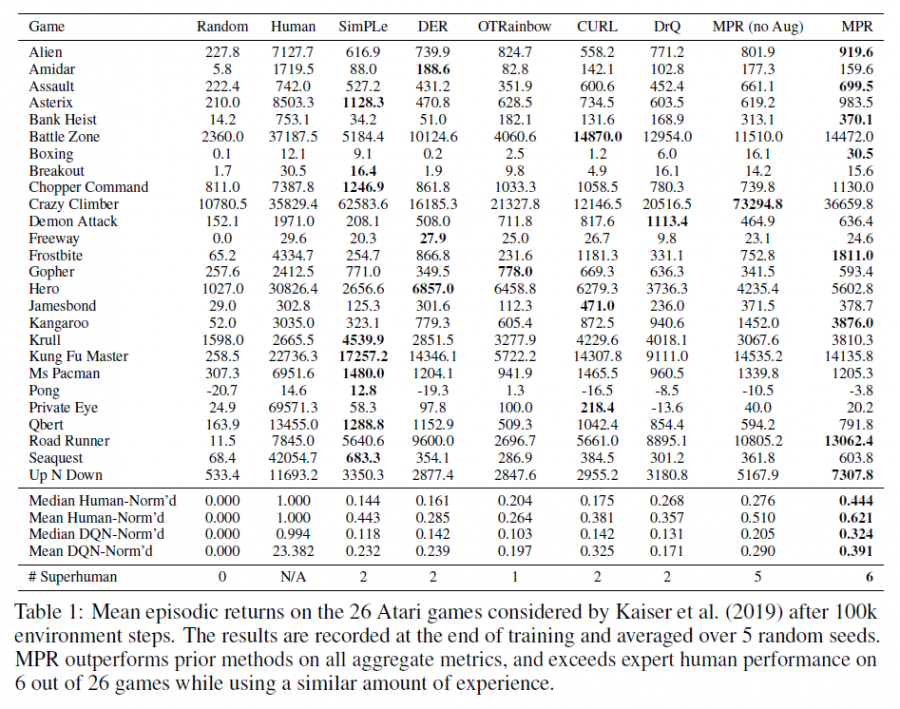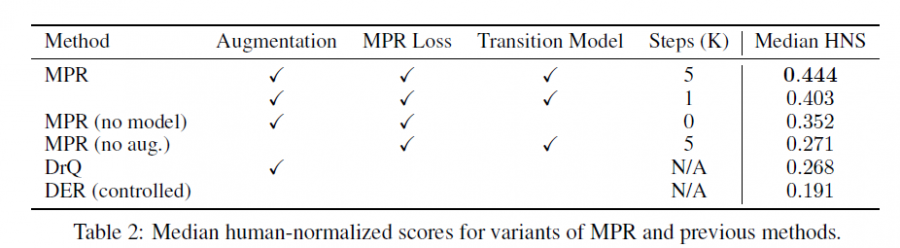# 单智能体强化学习算法

## Data-Efficient Reinforcement Learning with Momentum Predictive Representations

Posted by MY on August 27, 2020

## 二、解法

### 2.1 整体框架

MPR的整体方法框架如下图所示：MPR主要包括四个组件：online and momentum encoder、Transition Model、Projection Heads和Prediction Loss

#### 2.1.1 online and momentum encoder

online and momentum encoder分别用于构造类似于contrastive中的锚定数据和正样本。假设$\left(s_{t: t+K}, a_{t: t+K}\right)$是从buffer里采样得到的$K+1$个状态动作对，其中$K$是想要预测未来的step数。online encoder $f_{o}$用于将state $s_{t}$转换为表征$z_{t} \triangleq f_{o}\left(s_{t}\right)$，而momentum encoder $f_{m}$采用何凯明的MoCO的方式进行参数更新$\theta_{\mathrm{m}} \leftarrow \tau \theta_{\mathrm{m}}+(1-\tau) \theta_{\mathrm{o}}$，不经过梯度下降更新

#### 2.1.2 Transition Model

$\hat{y}_{t+k} \triangleq q\left(g_{o}\left(\hat{z}_{t+k}\right)\right), \forall \hat{z}_{t+k} \in \hat{z}_{t+1: t+K} ; \quad \tilde{y}_{t+k} \triangleq g_{m}\left(\tilde{z}_{t+k}\right), \forall \tilde{z}_{t+k} \in \tilde{z}_{t+1: t+K}$

#### 2.1.4 Prediction Loss

$\mathcal{L}^{\mathrm{MPR}}\left(s_{t: t+K}, a_{t: t+K}\right)=-\sum_{k=1}^{K}\left(\frac{\tilde{y}_{t+k}}{\left\|\tilde{y}_{t+k}\right\|_{2}}\right)^{\top}\left(\frac{\hat{y}_{t+k}}{\left\|\hat{y}_{t+k}\right\|_{2}}\right)$

$\mathcal{L}_{\theta}^{\text {total }}=\mathcal{L}_{\theta}^{\mathrm{RL}}+\lambda \mathcal{L}_{\theta}^{\mathrm{MPR}}$

### 2.2 算法流程## 三、实验内容## 四、缺点

• 思路还是局限于对状态做表征，对于RL本身的结构没有进行改进
• 严格来说本文不属于Contrastive Learning的范畴

## 五、优点

• 相比于之前的工作，增强对未来的预测更加提高了数据增强的效果